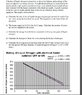# Heat energy and liquid nitrogen boil-off rate

## Homework Statement

Kindly refer to part (c). The woking should be power/0.35 (what I think according to the graph). But the answer is power (that is the answer of part b) divided by 0.02

## Homework Equations

I have used the ratio method simply

## The Attempt at a Solution

According to part (b), the amount of heat energy needed needed per second is 4.68 Joules (answer to part b). Now the gradient of the graph indicates that the mass loss per second is 0.35g/s. So we can imply the 4.68 Joules are needed to boil off 0.35g of liquid Nitrogen in one second. Then applying ratio, we can calculate for 1g. The correct answer to this question applies the same method but uses 0.02 instead of 0.35. Why is that so? Where did it come from?

#### Attachments

•BPho.jpg
54.3 KB · Views: 635